/
/
/
201) Find the direction in which the function increasing most
Not my Question
Flag Content

# Question : 201) Find the direction in which the function increasing most : 1757201

201) Find the direction in which the function is increasing most rapidly at the point P0.

f(x, y) = xey - ln(x), P0(-3, 0)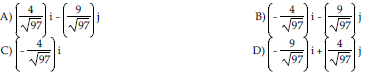202) Find the direction in which the function is increasing most rapidly at the point P0.

f(x, y, z) = xy - ln(z), P0(1, -2, 2)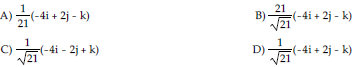203) Find the direction in which the function is increasing most rapidly at the point P0.

f(x, y, z) = x y2 + z2, P0(1, 1, 2)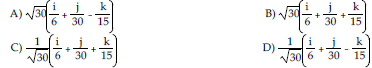204) Find the derivative of the function f(x, y) = x2 + xy + y2 at the point (-7, -6) in the direction in which the function increases most rapidly.

A)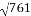B)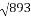C)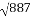D) 3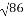205) Find the derivative of the function f(x, y) = x2 + xy + y2 at the point (6, 7) in the direction in which the function decreases most rapidly.

A) -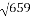B) -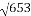C) -D) -3206) Find the derivative of the function f(x, y) = exy at the point (0, -5) in the direction in which the function increases most rapidly.

A) 15 B) 10 C) 5 D) 6

207) Find the derivative of the function f(x, y) = exy at the point (0, 3) in the direction in which the function decreases most rapidly.

A) -3 B) -9 C) -6 D) -2

208) Find the derivative of the function f(x, y) = tan-1 y/x at the point (4, -4) in the direction in which the function increases most rapidly.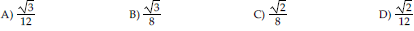209) Find the derivative of the function f(x, y) = tan-1 y/x at the point (5, -5) in the direction in which

the function decreases most rapidly.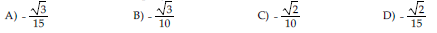210) Find the derivative of the function f(x, y, z) = ln(xy + yz + zx) at the point (5, 10, 15) in the direction in which the function increases most rapidly.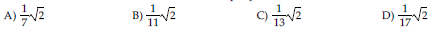## Solution 5 (1 Ratings )

Solved
Mathematics 3 Years Ago 50 Views